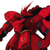# App Development

Announcements
Customer & Partners, DEC. 9, 11 AM ET: Qlik Product & Strategy Roadmap Session: Data Analytics REGISTER NOW
cancel
Showing results for
Did you mean:Creator

## Converting If-Statements into Set Analysis

Hey Qliksters,

I would like your assistance in converting some if-statements into set analysis if possible.

if(fruit = 'Apple', sum(field1)+sum(field2),

if(fruit='Orange', (sum(field1)+sum(field2))*2,

(sum(field1)+sum(field2)+sum(field3)*3)))

Is something like this possible to refactor into set analysis?

If-statements are slow, and I'd like to make my app faster by moving it to set analysis.

1 Solution

Accepted SolutionsLuminary Alumni

Set analysis is used mostly for selection, not conditional logic. If you'd like to avoid nested IFs then I'd change your statement to:

Pick(
WildMatch(fruit, 'Apple', 'Orange') + 1,
(sum(field1)+sum(field2)+sum(field3)) * 3,
sum(field1)+sum(field2),
(sum(field1)+sum(field2)) * 2
)

Explanation: Pick returns 1 if apple, 2 if orange, 0 if not matched.
Adding 1 will make it 2 if apple, 3 if orange, 1 if not matched.
Pick will select the item corresponding to the 1, 2 or 3 result.

So, it's essentially the equivalent of a Case or Switch statement.

Hope this helps.

2 RepliesLuminary Alumni

Set analysis is used mostly for selection, not conditional logic. If you'd like to avoid nested IFs then I'd change your statement to:

Pick(
WildMatch(fruit, 'Apple', 'Orange') + 1,
(sum(field1)+sum(field2)+sum(field3)) * 3,
sum(field1)+sum(field2),
(sum(field1)+sum(field2)) * 2
)

Explanation: Pick returns 1 if apple, 2 if orange, 0 if not matched.
Adding 1 will make it 2 if apple, 3 if orange, 1 if not matched.
Pick will select the item corresponding to the 1, 2 or 3 result.

So, it's essentially the equivalent of a Case or Switch statement.

Hope this helps.Creator
Author

Very clever indeed...

Thanks!Tags
Community Browser